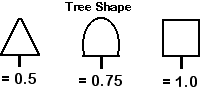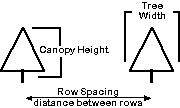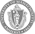## Block-Specific Sprayer Calibration Worksheet*

### Wesley R. Autio, Kathleen Leahy, and Jon ClementsBlock

### 1. CALCULATE DILUTE GALLONAGE REQUIREMENT PER ACRE (based on Tree Row Volume, TRV) = Dilute GPA

Tree Shape= (enter value from illustration above)

Tree Width= ft.

Canopy Height= ft.

Row Spacing= ft.

Dilute GPA= Dilute Gallons Per Acre

The formula: (Tree Shape X Tree Width (ft.) X Canopy Height (ft.) X 35) / Row Spacing (ft.)

Dilute Gallons Per Acre (GPA) is a fundamental concept of sprayer calibration. It is based Tree Row Volume (TRV), i.e. the total canopy volume (cubic feet) per acre and the fact (for Eastern orchards) it takes 1 gallon of spray material (water plus crop protectant) to cover 1,450 cubic feet of foliage. Dilute GPA should be calculated for every orchard block that differs significantly in tree size, shape, or age; and row spacing. The calculated GPA represents a full dilute (1X concentrate) spray volume of water plus crop protectant.

### 2. SPRAYER OUTPUT IF NOZZLED FOR SPECIFIC BLOCK = Sprayer Gallons per Minute (GPM)

Dilute GPA= Dilute Gallons Per Acre (calculated from #1., above)

Concentration (i.e., 2, 3 etc.)= X

Travel Speed= m.p.h.

Row Spacing= ft.

GPM= Gallons Per Minute

The formula: Dilute GPA/Concentration (1X, 2X, 3X etc.) = Gallons Per Acre; Gallons Per Acre X Travel Speed (m.p.h.) X Row Spacing (ft.) / 495 = Gallons Per Minute (GPM).

Gallons per minute (GPM) is the desired sprayer output (calibration 'target') based on dilute GPA, desired concentration (1X, 2X, 3X, etc.), tractor travel speed, and block row spacing. Dilute GPA and GPM are the two orchard factors essential to calibrate your sprayer to accurately deliver recommended pesticide rates on a block-by-block basis.

### 3. ADJUSTING FOR BLOCK DIFFERENCES WITH KNOWN SPRAYER OUTPUT

 GPM= Gallons per Minute Travel Speed= m.p.h. Row Spacing= ft. GPA= Gallons Per Acre Actual Concentration: Dilute GPA= Dilute Gallons Per Acre GPA= Gallons Per Acre Actual Concentration= X

The formula: Actual output per acre (GPA) = (GPM X 495)/Travel Speed X Row Spacing; Actual concentration = Dilute GPA/GPA

Given the known GPM (sprayer output), you can easily calculate GPA for another block with different row spacing and/or travel speed. (Of course, this assumes the same nozzle set-up.) Then divideDilute GPA by GPA to determine the actual concentration (2X, 3X, etc.). Ideally, dilute gallonage requirements per acre (based on TRV) and sprayer output(GPM) should be calculated first for each block.

#### *Some other sprayer calibration considerations:

• if tree size is variable, calibrate using average tree width/height measurements for the larger trees
• in general, two-thirds of the spray volume should be directed towards the top half of the tree; an exception is early in the season when it is reasonable to direct halk the spray in the bottom, half in the top (i.e., oil sprays)
• concentrate spraying is more sensitive to wind speed, drying conditions, and sprayer calibration errors; when spraying at 3X or more, the rate of crop protectant can be reduced by 20% from the recommended dilute rate for most insecticides and fungicides, except when the label indicates the product should be applied dilute only (usually needed for greater coverage); plant growth regulators should be applied as a dilute spray if possible. (Certainly no more concentrate than 3X)
• note that newer insecticide/fungicide/plant growth regulators will give a rate per acre only regardless of tree row volume and/or dilute vs. concentrate applications. In this case, use no less than the recommended rate per acre.
• always consult the label before making any pesticide application; the label is the law.

©Copyright 2009 . Site policies. Produced and maintained by the UMass Fruit Team.